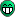# Fit with Heaviside function

Hello to all,

I am currently attempting to make a fit that would include the heaviside function, however any attempt so far has resulted in an error. Here is what I believed to be the closest to correct that I have managed:

``````TF1 *efit=new TF1("errorFit","*(x-)*(TMath::Erf((x-)/)))RooStats::Heaviside(x-)+",start, (start+a+b));

efit->SetParName(0,"t_0");
efit->SetParName(1,"Amplitude");
efit->SetParName(2,"Tau_r");
efit->SetParName(3,"Baseline");

efit->SetParameter(0,set_t0);
efit->SetParameter(1,amplitude);
efit->SetParameter(2,tau_r);
efit->SetParameter(3,baseline);

histo->Fit(efit,"RQ");
``````

The only real parameter than I need to vary is the variable t_0, however the others seem to cause problem (or maybe not and it is only the heaviside causing issue).

If anyone could provide help or insight into the problem that would be greatly appreciated.

Many thanks,

Ronan

Thank you, I took a look at the page, however I cannot find more information as to how I would write the heaside function into my fit function. When I run the line I have written above I get the following errors:

[quote]Error in TFormula::Compile: Bad numerical expression : "(x-)(TMath::Erf((x-)/)))RooStats::Heaviside(x-)"
Error in TF1::TF1: function: errorFit/
(x-)*(TMath::Erf((x-)/)))RooStats::Heaviside(x-)+ has 0 parameters instead of 1
Error in : function is zombie[/quote]

I know that the Erf works fine since it returned no errors prior to inserting the heaviside function.

Many thanks for future help and advice.

You should have noticed by now that RooStats::Heaviside is a class, not a mathematical function: [url]Are Heaviside and DiracDelta functions defined in ROOT?
You might try something like this (but I don’t guarantee that it works): `TF1 *efit = new TF1("errorFit", "*(x-)*(TMath::Erf((x-)/))*(x> ? 1.0 : 0.0)+", start, (start+a+b));`

Hum… so then implementing that directly in the fit is impossible. Alright, I guess a simple work around is required.

Many thanks.

Ronan

double post, my apologies.

My hat goes off to you Pepe Le Pew, your suggestion works perfectly.

Thank you.

If it works, you can simplify it further: `TF1 *efit = new TF1("errorFit", "*(x> ? x- : 0.0)*TMath::Erf((x-)/)+", start, (start+a+b));`

Hi,

You can use the RooStats::Heaviside for fitting in ROOT (i.e. without using RooFit), you just need to create a TF1 from it. You can do it, as for all other RoOFit classes implementing the RooAbsReal interface, by using the
RooAbsReal::asTF method,
see root.cern.ch/root/html/RooAbsRea … sReal:asTF

Lorenzo

But you don’t really expect anybody to be able to follow your idea, do you.I know this is a really old topic, but I have not found a solution (using TF1) in any search, so I am posting here what worked for me.

You can use TMath::Sign function to make the TF1 as the following:

TF1 *myHeavi = new TF1(“myHeavi”,"(1+TMath::Sign(1,x))/2",-20,20)

And if you want the half-maximum as parameter, you can simply do:

TF1 *myHeavi = new TF1(“myHeavi”,"(1+TMath::Sign(1,x - ))/2",-20,20)

Here is the link for a good explanation of the Sign function:

I hope it helps, if there is a simpler solution please let me know.

Cheers